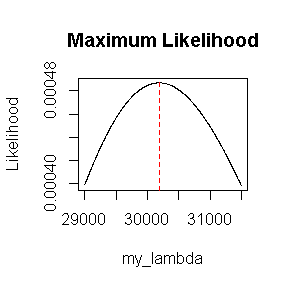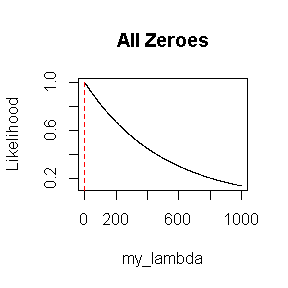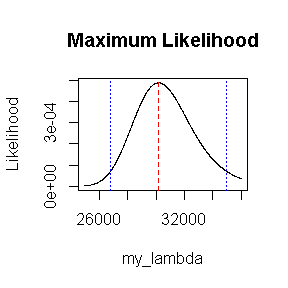APC: Aerobic Plate Count for Serial Dilutions

2019-03-20

The MPN package includes the apc() function to estimate the Aerobic Plate Count (APC) point estimate and confidence interval. The APC estimates the average density of colony forming units (CFUs) per milliliter. Although other techniques exist (1) to handle agar plate counts that are too-numerous-to-count (TNTC), apc() uses the maximum likelihood technique of Haas & Heller (2) and Haas et al. (3).

Maximum Likelihood Estimation

As with the Most Probable Number, the Aerobic Plate Count is estimated by maximizing likelihood. Using a modified version of the notation of Haas et al. (3), we write the likelihood function as:

$L = \prod_{i=1}^m \frac{{({\lambda}{V_i})} ^ {N_i}}{N_i!} {e ^ {-{\lambda}{V_i}}} \prod_{j=m+1}^n [1 - \Gamma(N_{L,j}-1, \lambda{V_j})]$

where

• $$\lambda$$ is the average microbial density (in CFU/ml) to be estimated
• $$m$$ is the number of scorable (countable) plates
• $$n$$ is the total number of plates
• $$N_i$$ is the CFU count at the $$i^{th}$$ scorable plate
• $$V_i$$ is the amount of inoculum (in ml) in the $$i^{th}$$ scorable plate
• $$N_{L,j}$$ is the CFU count limit in the $$j^{th}$$ plate above which the plate count is considered TNTC
• $$V_j$$ is the amount of inoculum (in ml) in the $$j^{th}$$ TNTC plate

As an R function:

L <- function(lambda, count, amount_scor, amount_tntc = NULL, tntc_limit = 100) {
#likelihood
scorable <- prod(dpois(count, lambda = lambda * amount_scor))
if (length(amount_tntc) > 0) {
incomplete_gamma <- pgamma(tntc_limit - 1, lambda * amount_tntc)
tntc <- prod(1 - incomplete_gamma)
return(scorable * tntc)
} else {
return(scorable)
}
}
L_vec <- Vectorize(L, "lambda")

apc() actually maximizes the log-likelihood function to solve for $$\hat{\lambda}$$, the maximum likelihood estimate (MLE) of $$\lambda$$ (i.e., the point estimate of APC). However, let’s demonstrate what is happening in terms of the likelihood function itself. Assume we start with four plates and 1 ml of undiluted inoculum. For the first two plates we use a 100-fold dilution; for the other two plates we use a 1,000-fold dilution. The first two plates were TNTC with limits of 300 and 250. The other plates had CFU counts of 28 and 20:

#APC calculation
library(MPN)
my_count       <- c(28, 20)          #Ni
my_amount_scor <- 1 * c(.001, .001)  #Vi
my_amount_tntc <- 1 * c(.01, .01)    #Vj
my_tntc_limit  <- c(300, 250)        #NLj
(my_apc <- apc(my_count, my_amount_scor, my_amount_tntc, my_tntc_limit))
#> $APC #>  30183.83 #> #>$conf_level
#>  0.95
#>
#> $LB #>  26792.25 #> #>$UB
#>  34963.34

If we plot the likelihood function, we see that $$\hat{\lambda}$$ maximizes the likelihood:

my_apc$APC #>  30183.83 my_lambda <- seq(29000, 31500, length = 1000) my_L <- L_vec(my_lambda, my_count, my_amount_scor, my_amount_tntc, my_tntc_limit) plot(my_lambda, my_L, type = "l", ylab = "Likelihood", main = "Maximum Likelihood") abline(v = my_apc$APC, lty = 2, col = "red")If all of the plates have zero counts, the MLE is zero:

all_zero <- c(0, 0)  #Ni
(apc_all_zero <- apc(all_zero, my_amount_scor)$APC) #>  0 my_lambda <- seq(0, 1000, length = 1000) L_all_zero <- L_vec(my_lambda, all_zero, my_amount_scor) plot(my_lambda, L_all_zero, type = "l", ylab = "Likelihood", main = "All Zeroes") abline(v = apc_all_zero, lty = 2, col = "red")Confidence Intervals apc() computes the confidence interval of $$\lambda$$ using the likelihood ratio approach described in Haas et al. (3). However, since this approach relies on large-sample theory, the results are more reliable for larger experiments. my_count <- c(28, 20) my_amount_scor <- 1 * c(.001, .001) my_amount_tntc <- 1 * c(.01, .01) my_tntc_limit <- c(300, 250) (my_apc <- apc(my_count, my_amount_scor, my_amount_tntc, my_tntc_limit)) #>$APC
#>  30183.83
#>
#> $conf_level #>  0.95 #> #>$LB
#>  26792.25
#>
#> $UB #>  34963.34 my_lambda <- seq(25000, 36000, length = 1000) my_L <- L_vec(my_lambda, my_count, my_amount_scor, my_amount_tntc, my_tntc_limit) plot(my_lambda, my_L, type = "l", ylab = "Likelihood", main = "Maximum Likelihood") abline(v = my_apc$APC, lty = 2, col = "red")
abline(v = my_apc$LB, lty = 3, col = "blue") abline(v = my_apc$UB, lty = 3, col = "blue")